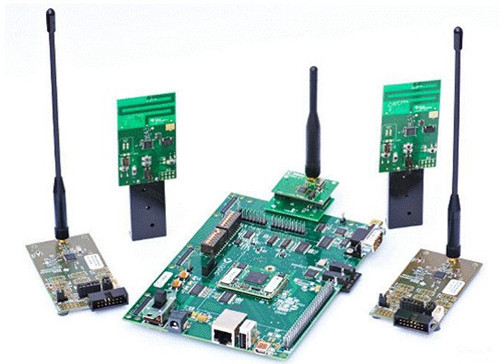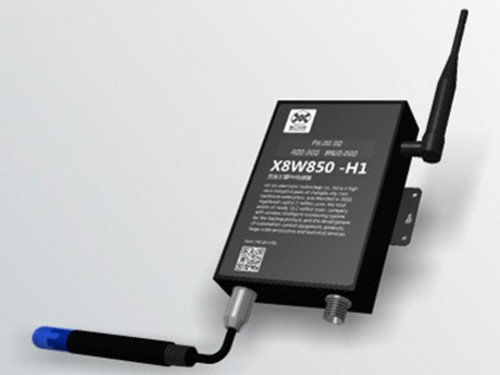|

# 无线传感器应用 传感器类型

无线传感器是当前信息领域中研究的热点之一，可用于特殊环境实现信号的采集、处理和发送。无线传感器网络是一种全新的信息获取和处理技术，在现实生活中得到了越来越广泛的应用。接下来小编为大家介绍无线传感器应用及传感器类型。无线传感器应用

1、军事领域的应用

在军事领域，由于WSN具有密集型、随机分布的特点，使其非常适合应用于恶劣的战场环境。利用WSN能够实现监测敌军区域内的兵力和装备、实时监视战场状况、定位目标、监测核攻击或者生物化学攻击等。

2、辅助农业生产

WSN特别适用于以下方面的生产和科学研究。例如，大棚种植室内及土壤的温度、湿度、光照监测、珍贵经济作物生长规律分析与测量、葡萄优质育种和生产等，可为农村发展与农民增收带来极大的帮助。采用WSN建设农业环境自动监测系统，用一套网络设备完成风、光、水、电、热和农药等的数据采集和环境控制，可有效提高农业集约化生产程度，提高农业生产种植的科学性。

3、生态监测与灾害预警

WSN可以广泛地应用于生态环境监测、生物种群研究、气象和地理研究、洪水、火灾监测。环境监测为环境保护提供科学的决策依据，是生态保护的基础。在野外地区或者不宜人工监测的区域布置WSN可以进行长期无人值守的不间断监测，为生态环境的保护和研究提供实时的数据资料。

具体的应用包括：通过跟踪珍稀鸟类等动物的栖息、觅食习惯进行濒危种群的研究;在河流沿线区域布置传感器节点，随时监测水位及水资源被污染的情况;在泥石流、滑坡等自然灾害容易发生的地区布置节点，可提前发出灾害预警，及时采取相应抗灾措施;可在重点保护林区布置大量节点随时监控内部火险情况，一旦发现火情，可立刻发出警报，并给出具体位置及当前火势的大小;可将节点布置在发生地震、水灾等灾害的地区、边远山区或偏僻野外地区，用于临时应急通信。

4、基础设施状态监测系统

WSN技术对于大型工程的安全施工以及建筑物安全状况的监测有积极的帮助作用。通过布置传感器节点，可以及时准确地观察大楼、桥梁和其他建筑物的状况，及时发现险情，及时进行维修，避免造成严重后果。

5、工业领域的应用

在工业安全方面，传感器网络技术可用于危险的工作环境，例如在煤矿、石油钻井、核电厂和组装线布置传感器节点，可以随时监测工作环境的安全状况，为工作人员的安全提供保证。另外，传感器节点还可以代替部分工作人员到危险的环境中执行任务，不仅降低了危险程度，还提高了对险情的反应精度和速度。

6、在智能交通中保障安全畅通

智能交通系统（ITS）是在传统交通体系的基础上发展起来的新型交通系统，它将信息、通信、控制和计算机技术以及其他现代通信技术综合应用于交通领域，并将“人—车—路—环境”有机地结合在一起。在现有的交通设施中增加一种无线传感器网络技术，将能够从根本上缓解困扰现代交通的安全、通畅、节能和环保等问题，同时还可以提高交通工作效率。因此，将无线传感器网络技术应用于智能交通系统已经成为近几年的研究热点。

7、在医疗系统大有作为

近年来，无线传感器网络在医疗系统和健康护理方面已有很多应用，例如，监测人体的各种生理数据，跟踪和监控医院中医生和患者的行动，以及医院的药物管理等。如果在住院病人身上安装特殊用途的传感器节点，例如心率和血压监测设备，医生就可以随时了解被监护病人的病情，在发现异常情况时能够迅速抢救。

8、促进信息家电设备更加智能

无线传感器网络的逐渐普及，促进了信息家电、网络技术的快速发展，家庭网络的主要设备已由单一机向多种家电设备扩展，基于无线传感器网络的智能家居网络控制节点为家庭内、外部网络的连接及内部网络之间信息家电和设备的连接提供了一个基础平台。传感器类型

1、振动传感器

每个节点的最高采样率可设置为4KHz，每个通道均设有抗混叠低通滤波器。采集的数据既可以实时无线传输至计算机，也可以存储在节点内置的2M数据存储器内，保证了采集数据的准确性。有效室外通讯距离可达300m，节点功耗仅30mA，使用内置的可充电电池，可连续测量18小时。如果选择带有USB接口的节点，您既可以通过USB接口对节点充电，也可以快速地把存储器内的数据下载到计算机里面。

2、应变传感器

节点结构紧凑，体积小巧，由电源模块、采集处理模块、无线收发模块组成，封装在PPS塑料外壳内。节点每个通道内置有独立的高精度120-1000Ω桥路电阻和放大调理电路，可以方便地由软件自动切换选择1/4桥，半桥，全桥测量方式，兼容各种类型的桥路传感器，比如应变，载荷，扭距，位移，加速度，压力，温度等。节点同时支持2线和3线输入方式，桥路自动配平，也可以存储在节点内置的2M数据存储器。有效室外通讯距离可达300 m。可连续测量十几个小时。

3、扭矩传感器

节点结构紧凑，体积小巧，封装在树脂外壳内。节点每个通道内置有高精度120-1000Ω桥路电阻和放大调理电路。桥路自动配平。节点的空中传输速率可以达到250K BPS，有效实时数据传输率达到4K SPS，有效室内通讯距离可达100米。节点设计有专门的电源管理软硬件，在实时不间断传输情况下，节点功耗仅25mA，使用普通9V电池，可连续测量几十个小时。对于长期监测应用，以5分钟间隔发送一次扭矩值，数年不需要更换电池，大大提高了系统的免维护性。

编辑总结：关于无线传感器应用及传感器类型就介绍到这里了，希望对大家有所帮助。想了解更多相识，可以关注网资讯。

`声明：本文由入驻焦点开放平台的作者撰写，除焦点官方账号外，观点仅代表作者本人，不代表焦点立场错误信息举报电话： 400-099-0099，邮箱：jubao@vip.sohu.com，或点此进行意见反馈，或点此进行举报投诉。`A B C D E F G H J K L M N P Q R S T W X Y Z
A - B - C - D - E
• A
• 鞍山
• 安庆
• 安阳
• 安顺
• 安康
• 澳门
• B
• 北京
• 保定
• 包头
• 巴彦淖尔
• 本溪
• 蚌埠
• 亳州
• 滨州
• 北海
• 百色
• 巴中
• 毕节
• 保山
• 宝鸡
• 白银
• 巴州
• C
• 承德
• 沧州
• 长治
• 赤峰
• 朝阳
• 长春
• 常州
• 滁州
• 池州
• 长沙
• 常德
• 郴州
• 潮州
• 崇左
• 重庆
• 成都
• 楚雄
• 昌都
• 慈溪
• 常熟
• D
• 大同
• 大连
• 丹东
• 大庆
• 东营
• 德州
• 东莞
• 德阳
• 达州
• 大理
• 德宏
• 定西
• 儋州
• 东平
• E
• 鄂尔多斯
• 鄂州
• 恩施
F - G - H - I - J
• F
• 抚顺
• 阜新
• 阜阳
• 福州
• 抚州
• 佛山
• 防城港
• G
• 赣州
• 广州
• 桂林
• 贵港
• 广元
• 广安
• 贵阳
• 固原
• H
• 邯郸
• 衡水
• 呼和浩特
• 呼伦贝尔
• 葫芦岛
• 哈尔滨
• 黑河
• 淮安
• 杭州
• 湖州
• 合肥
• 淮南
• 淮北
• 黄山
• 菏泽
• 鹤壁
• 黄石
• 黄冈
• 衡阳
• 怀化
• 惠州
• 河源
• 贺州
• 河池
• 海口
• 红河
• 汉中
• 海东
• 怀来
• I
• J
• 晋中
• 锦州
• 吉林
• 鸡西
• 佳木斯
• 嘉兴
• 金华
• 景德镇
• 九江
• 吉安
• 济南
• 济宁
• 焦作
• 荆门
• 荆州
• 江门
• 揭阳
• 金昌
• 酒泉
• 嘉峪关
K - L - M - N - P
• K
• 开封
• 昆明
• 昆山
• L
• 廊坊
• 临汾
• 辽阳
• 连云港
• 丽水
• 六安
• 龙岩
• 莱芜
• 临沂
• 聊城
• 洛阳
• 漯河
• 娄底
• 柳州
• 来宾
• 泸州
• 乐山
• 六盘水
• 丽江
• 临沧
• 拉萨
• 林芝
• 兰州
• 陇南
• M
• 牡丹江
• 马鞍山
• 茂名
• 梅州
• 绵阳
• 眉山
• N
• 南京
• 南通
• 宁波
• 南平
• 宁德
• 南昌
• 南阳
• 南宁
• 内江
• 南充
• P
• 盘锦
• 莆田
• 平顶山
• 濮阳
• 攀枝花
• 普洱
• 平凉
Q - R - S - T - W
• Q
• 秦皇岛
• 齐齐哈尔
• 衢州
• 泉州
• 青岛
• 清远
• 钦州
• 黔南
• 曲靖
• 庆阳
• R
• 日照
• 日喀则
• S
• 石家庄
• 沈阳
• 双鸭山
• 绥化
• 上海
• 苏州
• 宿迁
• 绍兴
• 宿州
• 三明
• 上饶
• 三门峡
• 商丘
• 十堰
• 随州
• 邵阳
• 韶关
• 深圳
• 汕头
• 汕尾
• 三亚
• 三沙
• 遂宁
• 山南
• 商洛
• 石嘴山
• T
• 天津
• 唐山
• 太原
• 通辽
• 铁岭
• 泰州
• 台州
• 铜陵
• 泰安
• 铜仁
• 铜川
• 天水
• 天门
• W
• 乌海
• 乌兰察布
• 无锡
• 温州
• 芜湖
• 潍坊
• 威海
• 武汉
• 梧州
• 渭南
• 武威
• 吴忠
• 乌鲁木齐
X - Y - Z
• X
• 邢台
• 徐州
• 宣城
• 厦门
• 新乡
• 许昌
• 信阳
• 襄阳
• 孝感
• 咸宁
• 湘潭
• 湘西
• 西双版纳
• 西安
• 咸阳
• 西宁
• 仙桃
• 西昌
• Y
• 运城
• 营口
• 盐城
• 扬州
• 鹰潭
• 宜春
• 烟台
• 宜昌
• 岳阳
• 益阳
• 永州
• 阳江
• 云浮
• 玉林
• 宜宾
• 雅安
• 玉溪
• 延安
• 榆林
• 银川
• Z
• 张家口
• 镇江
• 舟山
• 漳州
• 淄博
• 枣庄
• 郑州
• 周口
• 驻马店
• 株洲
• 张家界
• 珠海
• 湛江
• 肇庆
• 中山
• 自贡
• 资阳
• 遵义
• 昭通
• 张掖
• 中卫

1室1厅1厨1卫1阳台

1
2
3
4
5

0
1
2

1

1

0
1
2
3报名成功，资料已提交审核A B C D E F G H J K L M N P Q R S T W X Y Z
A - B - C - D - E
• A
• 鞍山
• 安庆
• 安阳
• 安顺
• 安康
• 澳门
• B
• 北京
• 保定
• 包头
• 巴彦淖尔
• 本溪
• 蚌埠
• 亳州
• 滨州
• 北海
• 百色
• 巴中
• 毕节
• 保山
• 宝鸡
• 白银
• 巴州
• C
• 承德
• 沧州
• 长治
• 赤峰
• 朝阳
• 长春
• 常州
• 滁州
• 池州
• 长沙
• 常德
• 郴州
• 潮州
• 崇左
• 重庆
• 成都
• 楚雄
• 昌都
• 慈溪
• 常熟
• D
• 大同
• 大连
• 丹东
• 大庆
• 东营
• 德州
• 东莞
• 德阳
• 达州
• 大理
• 德宏
• 定西
• 儋州
• 东平
• E
• 鄂尔多斯
• 鄂州
• 恩施
F - G - H - I - J
• F
• 抚顺
• 阜新
• 阜阳
• 福州
• 抚州
• 佛山
• 防城港
• G
• 赣州
• 广州
• 桂林
• 贵港
• 广元
• 广安
• 贵阳
• 固原
• H
• 邯郸
• 衡水
• 呼和浩特
• 呼伦贝尔
• 葫芦岛
• 哈尔滨
• 黑河
• 淮安
• 杭州
• 湖州
• 合肥
• 淮南
• 淮北
• 黄山
• 菏泽
• 鹤壁
• 黄石
• 黄冈
• 衡阳
• 怀化
• 惠州
• 河源
• 贺州
• 河池
• 海口
• 红河
• 汉中
• 海东
• 怀来
• I
• J
• 晋中
• 锦州
• 吉林
• 鸡西
• 佳木斯
• 嘉兴
• 金华
• 景德镇
• 九江
• 吉安
• 济南
• 济宁
• 焦作
• 荆门
• 荆州
• 江门
• 揭阳
• 金昌
• 酒泉
• 嘉峪关
K - L - M - N - P
• K
• 开封
• 昆明
• 昆山
• L
• 廊坊
• 临汾
• 辽阳
• 连云港
• 丽水
• 六安
• 龙岩
• 莱芜
• 临沂
• 聊城
• 洛阳
• 漯河
• 娄底
• 柳州
• 来宾
• 泸州
• 乐山
• 六盘水
• 丽江
• 临沧
• 拉萨
• 林芝
• 兰州
• 陇南
• M
• 牡丹江
• 马鞍山
• 茂名
• 梅州
• 绵阳
• 眉山
• N
• 南京
• 南通
• 宁波
• 南平
• 宁德
• 南昌
• 南阳
• 南宁
• 内江
• 南充
• P
• 盘锦
• 莆田
• 平顶山
• 濮阳
• 攀枝花
• 普洱
• 平凉
Q - R - S - T - W
• Q
• 秦皇岛
• 齐齐哈尔
• 衢州
• 泉州
• 青岛
• 清远
• 钦州
• 黔南
• 曲靖
• 庆阳
• R
• 日照
• 日喀则
• S
• 石家庄
• 沈阳
• 双鸭山
• 绥化
• 上海
• 苏州
• 宿迁
• 绍兴
• 宿州
• 三明
• 上饶
• 三门峡
• 商丘
• 十堰
• 随州
• 邵阳
• 韶关
• 深圳
• 汕头
• 汕尾
• 三亚
• 三沙
• 遂宁
• 山南
• 商洛
• 石嘴山
• T
• 天津
• 唐山
• 太原
• 通辽
• 铁岭
• 泰州
• 台州
• 铜陵
• 泰安
• 铜仁
• 铜川
• 天水
• 天门
• W
• 乌海
• 乌兰察布
• 无锡
• 温州
• 芜湖
• 潍坊
• 威海
• 武汉
• 梧州
• 渭南
• 武威
• 吴忠
• 乌鲁木齐
X - Y - Z
• X
• 邢台
• 徐州
• 宣城
• 厦门
• 新乡
• 许昌
• 信阳
• 襄阳
• 孝感
• 咸宁
• 湘潭
• 湘西
• 西双版纳
• 西安
• 咸阳
• 西宁
• 仙桃
• 西昌
• Y
• 运城
• 营口
• 盐城
• 扬州
• 鹰潭
• 宜春
• 烟台
• 宜昌
• 岳阳
• 益阳
• 永州
• 阳江
• 云浮
• 玉林
• 宜宾
• 雅安
• 玉溪
• 延安
• 榆林
• 银川
• Z
• 张家口
• 镇江
• 舟山
• 漳州
• 淄博
• 枣庄
• 郑州
• 周口
• 驻马店
• 株洲
• 张家界
• 珠海
• 湛江
• 肇庆
• 中山
• 自贡
• 资阳
• 遵义
• 昭通
• 张掖
• 中卫• 手机• 分享
• 设计
免费设计
• 计算器
装修计算器
• 入驻
合作入驻
• 联系
联系我们
• 置顶
返回顶部